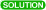Problems & Puzzles: Problems

1.- David Silverman Question2.- Carl Pomerance Observation

3.- Erdos Conjecture

4.- Fortune Conjecture

5.- Missed prime factors in Woodall numbers

6.- Primes and Partitions7.- Primes and Parity of x and its inverse x’, module p

8.-(m, n+1) and (m+1, n) with the same set of prime factors

9.-Let f(n) be the number of ways of representing n10.-Find a 500-digit prime p and positive integers x, y

11.-Here you are asked to find primes of the following form (pr - 1)/(pd -1), expressible in more than one way.

12.-Prime producing polynomials

13.-The Gap of the Beast14.-N and N^2-1 powerful numbers

15.-Why the Twin Constant is so close to 2/3?

16.-A ‘little puzzle’ from Mike Keith (12/12/98)21.- Divisors (III). Certain questions about s(N), the sum of divisors of a number N.

22.- Divisors (IV) Aliquot sequences, factorizing s1131 (276)

23.- Divisors (V) Aliquot sequences, Sociable Numbers.

24.- Carmichael Numbers

25.- The William Paulsen's Prime Numbers Maze

26.- The earliest Cunningham Chains.

27.- Congruent K contiguous products of consecutive numbers.

28.- K least consecutive numbers n such that are not squarefree

29.- Brier Numbers

30.- A stepladder to Ba sequence

31.- Fibonacci all composites sequence

32.- Fibonacci and permuted all composites sequences

33.- The extreme prime triplets

34.- Proving & hiding

35.- More wrong turns...

36.The Liskovets-Gallot numbers

37. The Liu Fengsui's Prime Formula

38.  Sebastián Martín Ruiz Prime formulas

39.  The primes sequence and the Sebastian's function

40.  Crump's equation

42 . Floor Exponent Prime Sequences

44 . Twin-primes producing polynomials race

45 . A follow up to Problem 8

46 . Holes and Crowds-I

47 . Holes and Crowds-II

56.  p1*p2*…*pk = q1+q2+…+qk

57.  Carmichael numbers c & 2c+1

59.  Wieferich-non-Wilson primes

60.  Symmetric primes on each side.

61. Problem 20 revisited

62. Symmetric k-tuples of consecutive primes

63. 3x3 Magic squares composed by triangular numbers.

65. Prime convex polygons

66. Every positive is integer is the sum of 3 palindromes. Looking for another proof.

68. More on Brier numbers.

69. More on Brier numbers-II.

72. Consecutive primes inside a 9x9 square such that...

73. Minimal symmetric tuples from consecutive twin primes...

76.  More on Egyptian fractions

77.  Accumulating primes in a 2-pans balance (A332788)

 Records   |  Conjectures  |  Problems  |  PuzzlesHome | Melancholia | Problems & Puzzles | References | News | Personal Page | Puzzlers | Search | Bulletin Board | Chat | Random Link Copyright © 1999-2012 primepuzzles.net. All rights reserved.